Home : Maplesoft Products : Maple 18 : New Features in Maple 18 : Statistics for Students

# Statistics for Students

The Student package is a collection of sub-packages designed to assist with the teaching and learning of standard undergraduate mathematics. For Maple 18, we added a new subpackage called Statistics to the Student family. Student[Statistics] provides more detailed explanations, instructions, and demonstrations about the material covered in statistics courses than is offered in the standard Statistics package.

With the Student Statistics package, students can work with data, visualize statistical distributions, and apply hypothesis tests. Students can even interactively explore the properties of different probability distributions.

There are many ways to interact with this new package. Typically, students will use Student[Statistics] to:

### Create Data Samples

There are three types of data samples valid in this package:

1. A data sample that follows a specific distribution
Data samples can easily be created using random variables with corresponding distributions. For example, to create a Normal random variable, one would call NormalRandomVariable(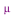,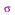).

2. A data sample stored in a list or a Vector
Each element in a list or Vector data sample represents a single recorded observation. There is no difference between a list sample and a Vector sample, either is valid.

3. A data sample stored in a Matrix
A Matrix data sample is treated as a collection of several list or Vector samples. Each column of the Matrix represents an individual sample.

### Work with Data Samples

1. Compute quantities of data samples
There are many commands for computing quantities of data samples. This includes many different quantities, such as the Mean Value, the Standard Deviation, the Skewness, and many more. Also in this package, users are not only able to query for a symbolic formula or exact numeric value for a given quantity from a data sample, but it is also possible to return a visualization of the result.

2. Explore distributions
Users can easily explore the important properties of a distribution by using the command, ExploreRV. ExploreRV takes an arbitrary statistical distribution and displays an interactive interface to explore its various parameters. This includes returning key quantities, such as the mean, median and more, as well as returning visualizations of the CDF and PDF.

3. Apply hypothesis tests
To test a given hypothesis, there are several hypothesis tests available, including OneSampleTTest, ChiSquareGoodnessOfFitTest, ShapiroWilkWTest, and more. To better explain how and when to use different hypothesis tests, a new command, TestsGuide, is introduced in this package to direct a student through the process of choosing an appropriate test.

### Examples

 >#### Example

We first define a discrete distribution:

 >Then we can study some properties of this distribution:

 >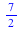>To return a numeric value, we need to specify the optional parameter numeric or numeric=true

 >We can set the optional parameter output to output=plot to see a plot demonstration.

 >>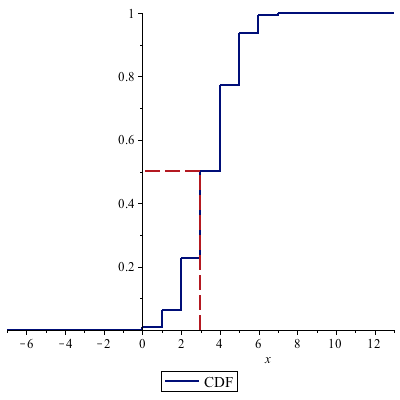To get the formula for computing the specific property of a distribution, we need to specify the optional parameter inert or inert=true

 >Try another distribution, which is continuous.

 >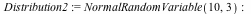>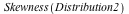>#### Example

Say we have observed and recorded some data. We can then put the data onto a list or Vector:

 >Compute the mode and the 30th percentile of this data sample:

 >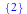>We can randomly generate a data sample from a known distribution with a specified sample size.

 >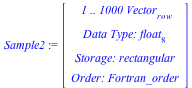Compare the data sample generated and the original distribution.

 >>>Then, test the sample to see if it follows the exponential distribution with parameter 5.

 >Chi-Square Test for Suitable Probability Model

 ---------------------------------------------- Null Hypothesis: Sample was drawn from specified probability distribution Alt. Hypothesis: Sample was not drawn from specified probability distribution Bins:                    32 Degrees of freedom:      31 Distribution:            ChiSquare(31) Computed statistic:      30.784 Computed pvalue:         0.477134 Critical value:          44.9853428040743 Result: [Accepted] There is no statistical evidence against the null hypothesis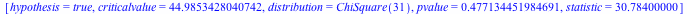#### Example

Create a Matrix data sample:

 >If we want to compute the mean value of this Matrix data sample, then we are going to compute the mean values of three list or Vector data samples stored in the columns correspondingly.

 >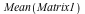To have both value and plot returned, specify the option output=both.

 >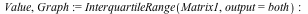>>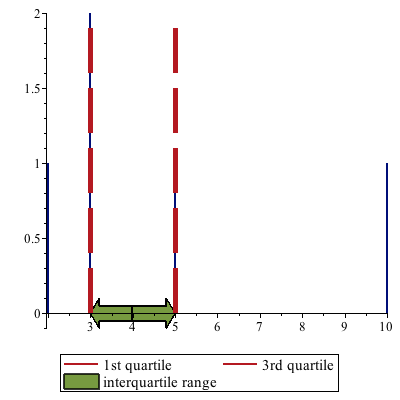#### Example

In our last example, we can use the command ExploreRV to explore some important properties of distributions.

 >Random Variables: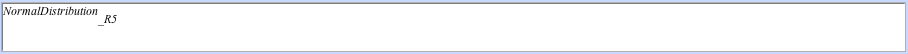Parameters: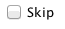Statistical Properties:

 MeanSupportMedianVariance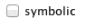Mode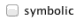Moment Generating FunctionProbability Distribution Function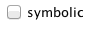Cumulative Distribution Function## Ready for the next step?

*Evaluations are not available for students or personal edition users.